Homework Help Question & Answers

# 1) Show that zo-0 is a regular singular point for the diferenta equation Zo = 0 is a regular sing...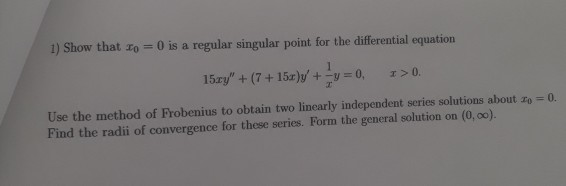please show the recurrence formula

1) Show that zo-0 is a regular singular point for the diferenta equation Zo = 0 is a regular singular point for the differential equation 15ェy" + (7 + 15r)y, +-y = 0, x>0. Use the method of Frobenius to obtain two linearly independent series solutions about zo Find the radii of convergence for these series. Form the general solution on (0, 0o). 0.

#### Homework Answers

Answer #1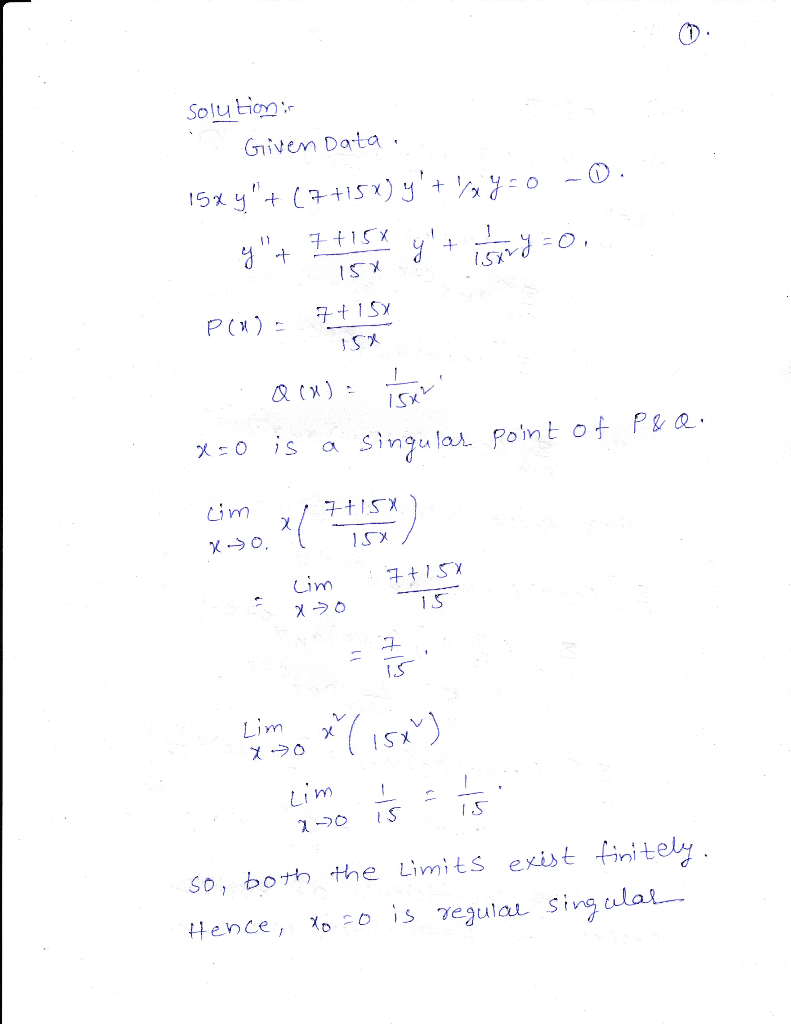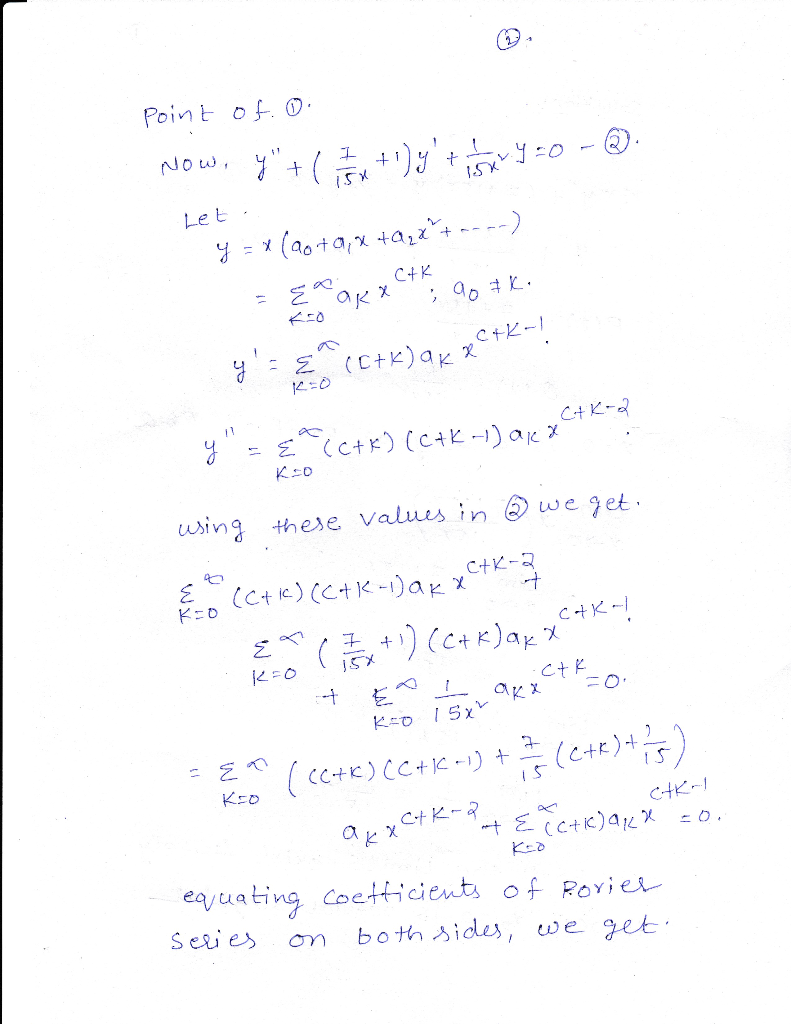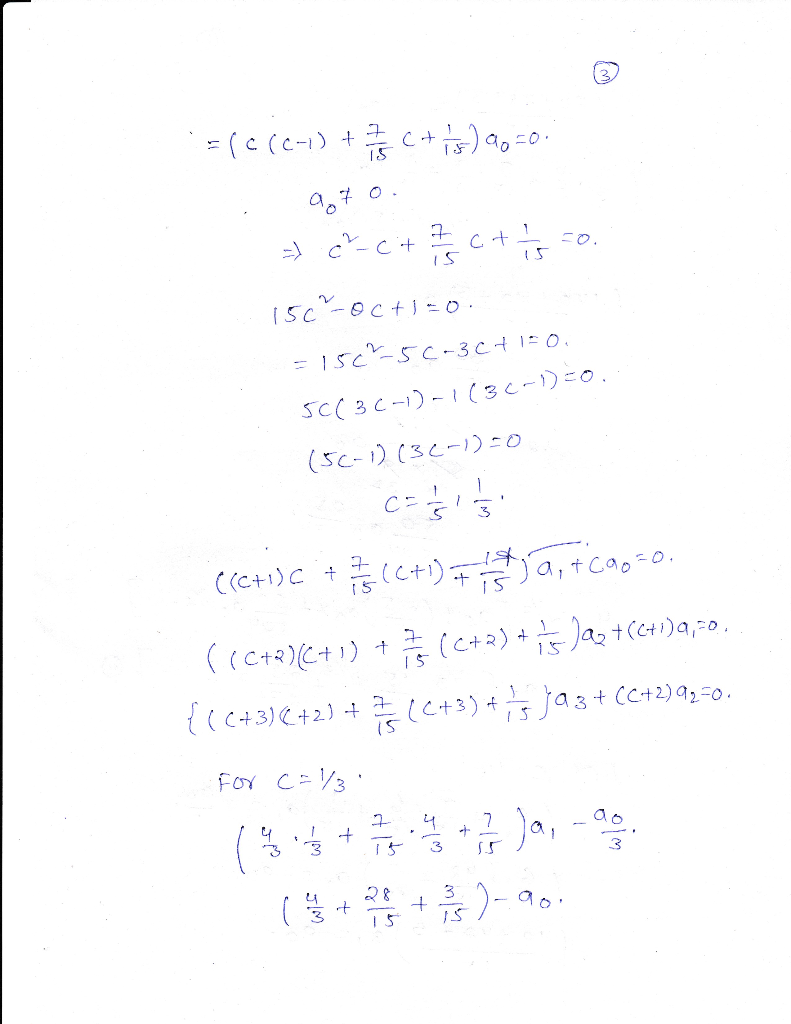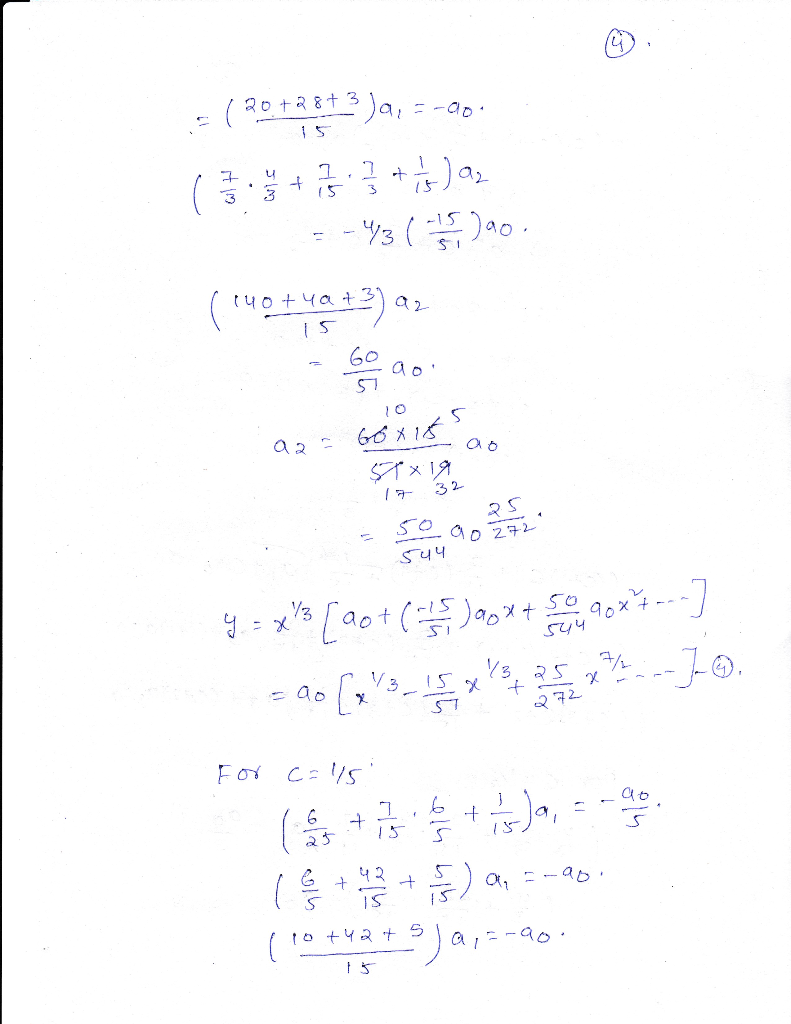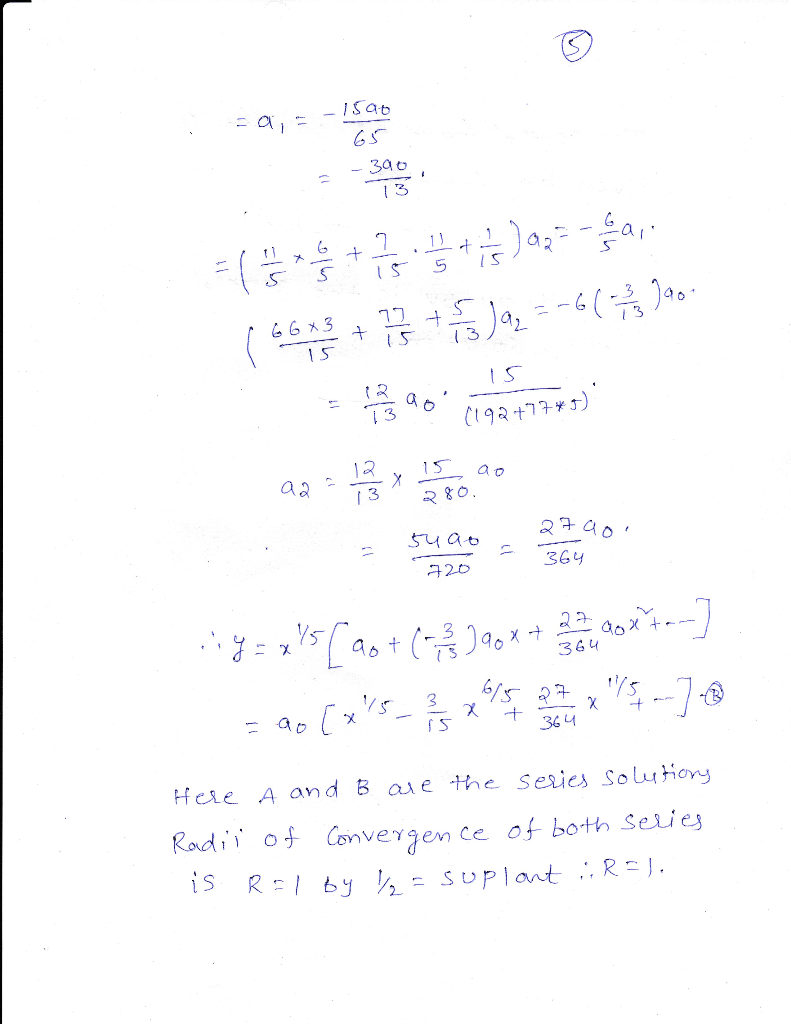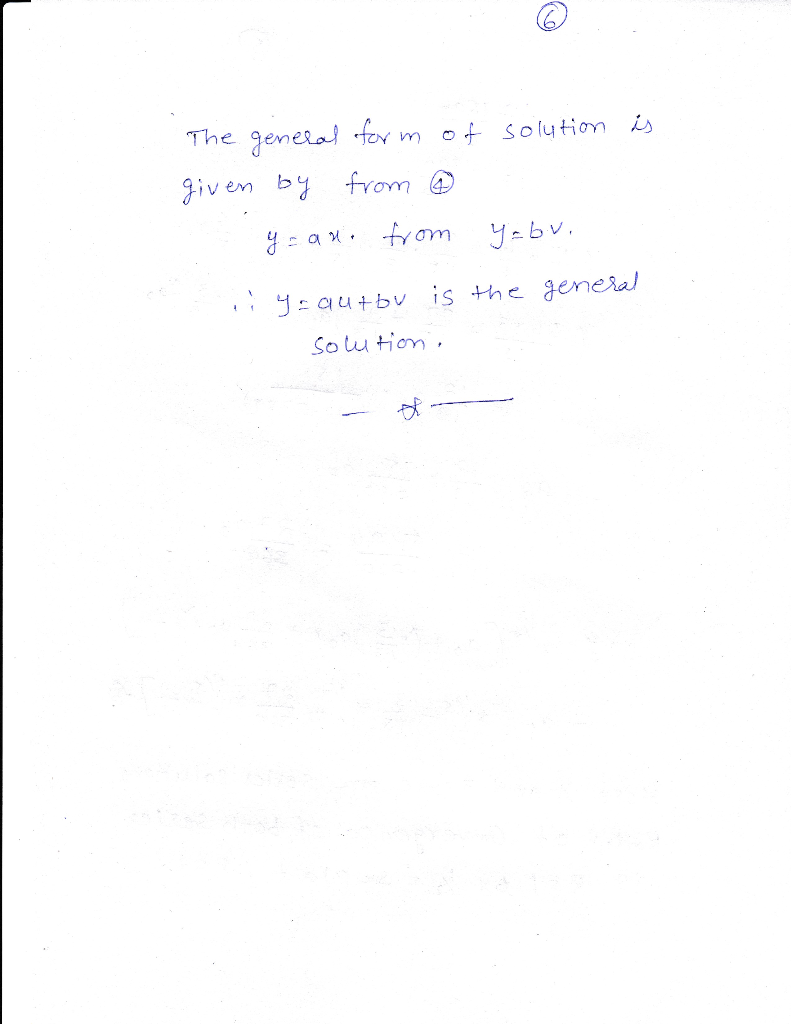Know the answer?
Your Answer:

#### Post as a guest

Your Name:

What's your source?

#### Earn Coin

Coins can be redeemed for fabulous gifts.

Not the answer you're looking for? Ask your own homework help question. Our experts will answer your question WITHIN MINUTES for Free.
Similar Homework Help Questions
• ### Given that x =0 is a regular singular point of the given differential equation, show that...

Given that x =0 is a regular singular point of the given differential equation, show that the indicial roots of the singularity do not differ by an integer. Use the method of Frobenius to obtain two linearly independent series solutions about x = 0. Form the general solution on (0, ∞) 2xy''-y'+y=0

• ### 4. Given that x =0 is a regular singular point of the given differential equation, show...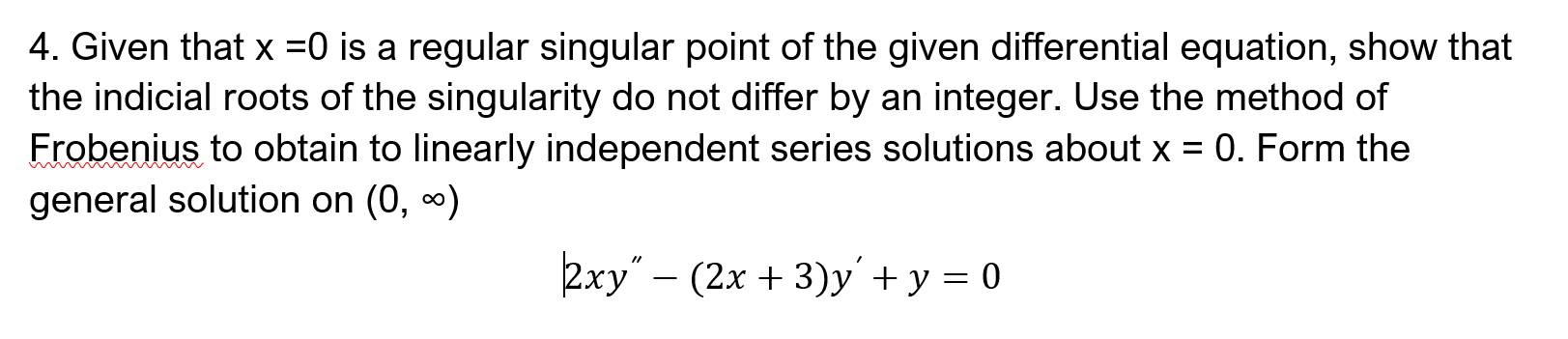4. Given that x =0 is a regular singular point of the given differential equation, show that the indicial roots of the singularity do not differ by an integer. Use the method of Frobenius to obtain to linearly independent series solutions about x = 0. Form the general solution on (0, 0) kxy” – (2x + 3)y' + y = 0

• ### 20. 0/2 points | Previous Answers ZillDiffEQ9 6.3.018 The point x 0 is a regular singular point o...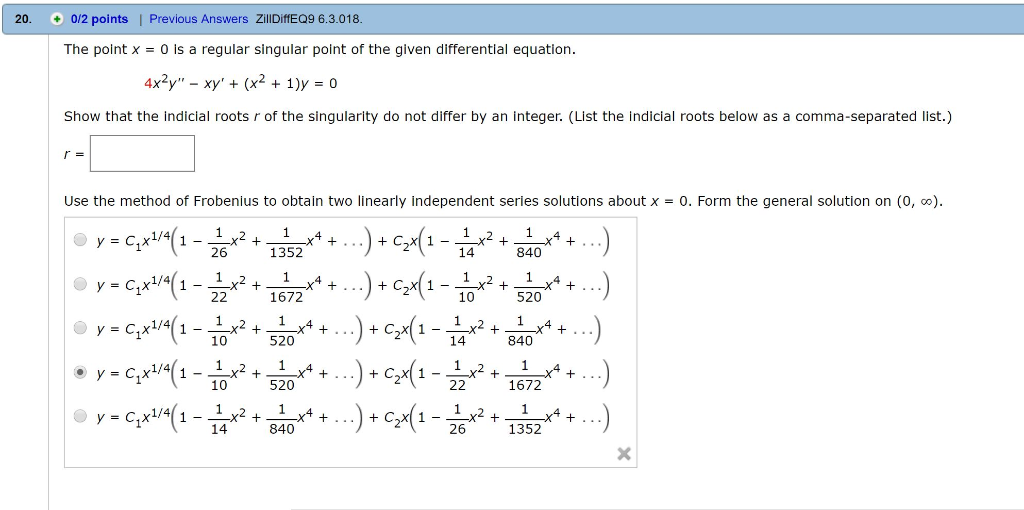20. 0/2 points | Previous Answers ZillDiffEQ9 6.3.018 The point x 0 is a regular singular point of the given differential equation. 4x2y"-xy + (x2 + 1)y = 0 Show that the indicial roots r of the singularity do not differ by an integer. (List the indicial roots below as a comma-separated list.) Use the method of Frobenius to obtain two linearly independent series solutions about x-0. Form the general solution on (0, ) 2015 340**. y = C-X1/4 1672...

• ### Consider the differential equation 4x2y′′ − 8x2y′ + (4x2 + 1)y = 0 (a) Verify that x0 = 0 is a regular singular point of...

Consider the differential equation 4x2y′′ − 8x2y′ + (4x2 + 1)y = 0 (a) Verify that x0 = 0 is a regular singular point of the differential equation and then find one solution as a Frobenius series centered at x0 = 0. The indicial equation has a single root with multiplicity two. Therefore the differential equation has only one Frobenius series solution. Write your solution in terms of familiar elementary functions. (b) Use Reduction of Order to find a second...

• ### 7. Consider the differential equation (a) Show that z 0 is a regular singular point of the above differential equation (b) Let y(x) be a solution of the differential equation, where r R and the s...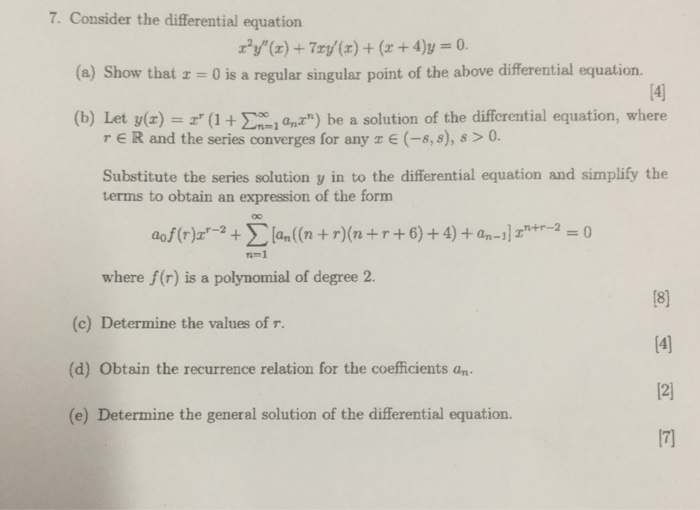7. Consider the differential equation (a) Show that z 0 is a regular singular point of the above differential equation (b) Let y(x) be a solution of the differential equation, where r R and the series converges for any E (-8,s), s > 0 Substitute the series solution y in to the differential equation and simplify the terms to obtain an expression of the form 1-1 where f(r) is a polynomial of degree 2. (c) Determine the values of r....

• ### Given: About a regular singular point x = 0, I have solved for and , I...

Given: About a regular singular point x = 0, I have solved for and , I have also solved for Therefore a.) I need to find the first three non-zero terms of the two linearly independent solutions using the GENERAL RECURRENCE FORMULA: Where NOT BY PLUGGING THE SERIES SOLUTION INTO THE DIFFERENTIAL EQUATION. Please show ALL your steps when using the formula, every value you plug in and how to do it for the first 3 terms ()

• ### 2. Solve each of these ODEs using power series method expanded around Xo = 0. Find...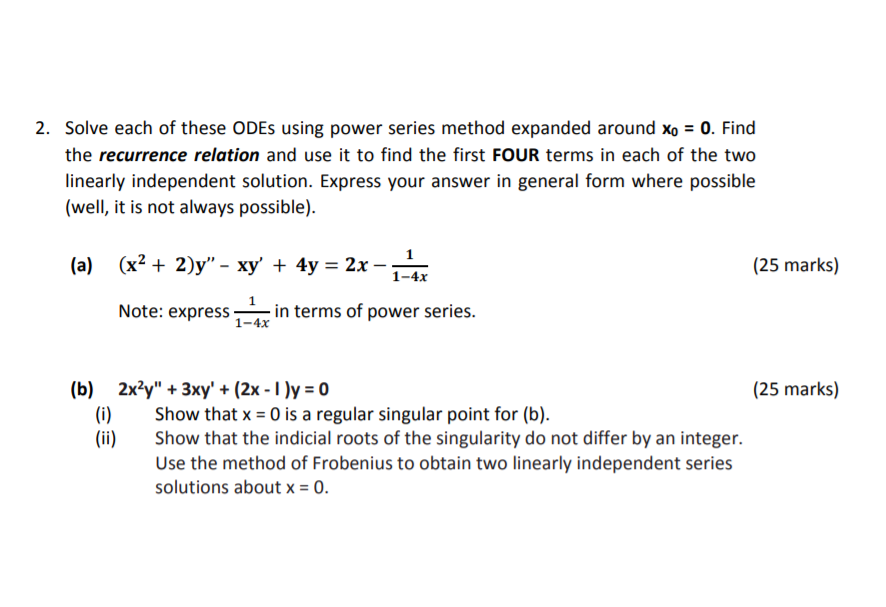2. Solve each of these ODEs using power series method expanded around Xo = 0. Find the recurrence relation and use it to find the first FOUR terms in each of the two linearly independent solution. Express your answer in general form where possible (well, it is not always possible). (a) (25 marks) (x2 + 2)y” - xy + 4y = 2x - 1-47 Note: expressa in terms of power series. (b) 2x2y" + 3xy' + (2x - 1) =...

• ### 1 point) Consider the differential equation which has a regular singular point at x = O. The indi...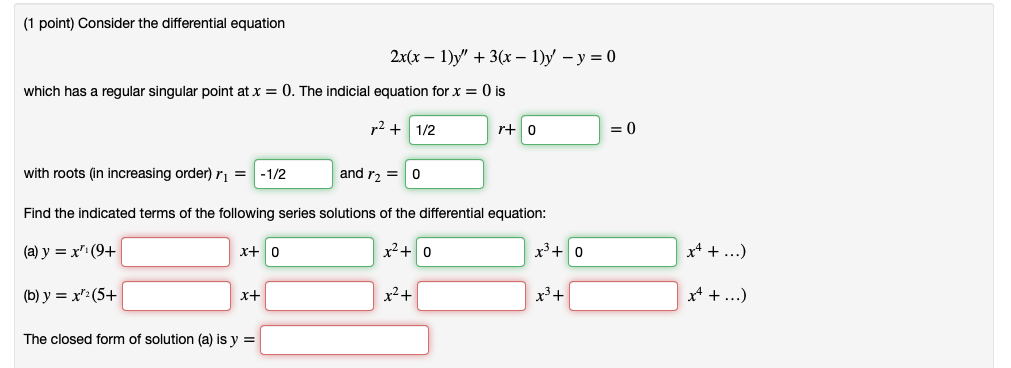1 point) Consider the differential equation which has a regular singular point at x = O. The indicial equation for x 0 is r1/2 r+ 0 =0 and r O with roots (in increasing order) r1/2 Find the indicated terms of the following series solutions of the differential equation: (a) y = x, (94 (b)y-x(5+ The closed form of solution (a) is y = xtO r3+ 1 point) Consider the differential equation which has a regular singular point at x...

• ### Advanced Engineering Mathematics (Zill), Chapter 5.2, Question 22

x2y(2)+xy(1)+(x2-4/9)y=0-y(2) means the second derivative of y. x=0 is a regular singular point of the given differential equation. Show that the indicial roots of the singularity do not differ by an integer. Use the method ofFrobenius to obtain two linearly independent series solutions about x=0. Form the general solution on (0,infinity).

• ### (1 point) Consider the differential equation 2x(x )y"3 - 1)y -y0 which has a regular singular...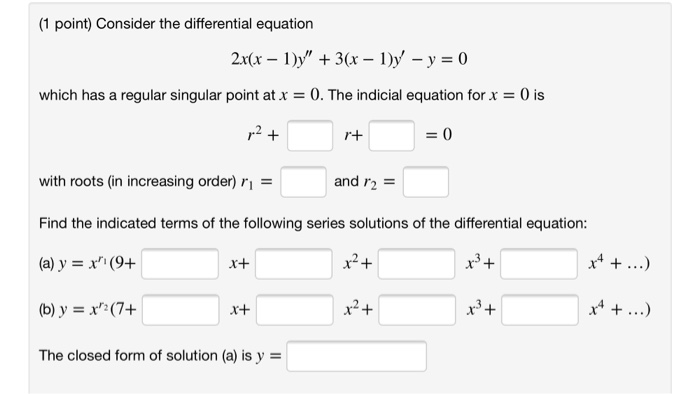(1 point) Consider the differential equation 2x(x )y"3 - 1)y -y0 which has a regular singular point atx 0. The indicial equation for x 0 is 2+ 0 r+ with roots (in increasing order) r and r2 Find the indicated terms of the following series solutions of the differential equation: x4. (a) y =x (9+ x+ (b) y x(7+ The closed form of solution (a) is y (1 point) Consider the differential equation 2x(x )y"3 - 1)y -y0 which has...

Free Homework App

Scan Your Homework
to Get Instant Free Answers
Need Online Homework Help?

Get Answers For Free
Most questions answered within 3 hours.